Published in: Izv. Ross. Akad. Nauk, Ser. Mat., 68 (1998), no. 6, pp. 27-52.;
English translation: Russian Acad. Sci. Math. Izv., 68 (1998), no. 6.

A B S T R A C T
B a b e n k o A. G.
Exact Jackson - Stechkin Inequality for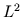- Approximations on the Segment with Jacobi Weight and on Projective Spaces
Let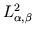be a Hilbert space of real functions on the segment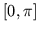with the inner product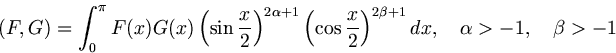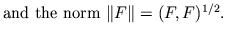In the paper had been proved that exact Jackson - Stechkin inequality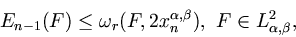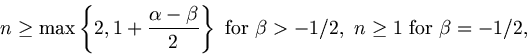among the best approximation of the function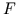by cosine polynomials of the order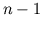and its generalized modulus of continuity of the (real) order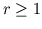is valid in the case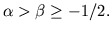Here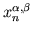is the first positive zero of Jacobi cosine polynomial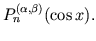Analogouse results for mean squared approximations of functions of many variables given on the projective spaces are deduced from here.

Bibliogr. 49 titles.